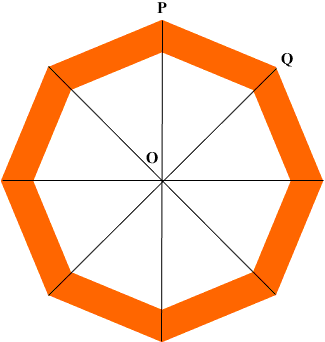Quandaries and Queries Hello, My son and I are making an octagon shaped bed frame. We are going nuts trying to figure out what angle to cut the boards to make an outline of an octagon. It seems that the 8 inside angles of the 8 "corners" are 120*, but what is the angle that the 2x6 wood should be cut so that they will angle together to form the outline of the octagon?  Thankz Trish Hi Trish, If you join each vertex of a regular octagon to to the center you get eight, congruent, isosceles triangles. One such triangle is POQ in the diagram below.Angle POQ then has measure 360/8= 45 degrees. Since POQ is isosceles, angles OQP and QPO are equal. But the sum of the angles in a triangle is 180 degrees and thus Angle QPO = (180 - 45)/2 = 67.5 degrees. Thus, if you cut each 2 by 6 at an angle of 67.5 degrees they will fit together to form a regular octagon. If you have a digital camera send us a photograph of the bed when you are done. Penny Go to Math Central